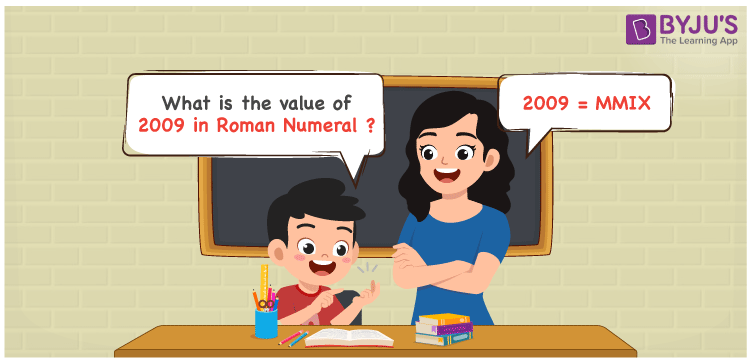# 2009 in Roman Numerals

2009 in Roman Numerals is MMIX. Any Roman numeral uses only the 7 primary symbols which are I, V, X, L, C, D, and M where M represents 1000, X represents 10 and I represents 1. MMXI follows the addition and subtraction rule of Roman numerals and it is the number 2009. What are Roman numerals? can be referred for more details. The details on how to write 2009 is given in the next section of this article.

 Number Roman Numeral 2009 MMIX

## How to Write 2009 in Roman Numerals?To convert 2009 in Roman Numerals, we need to represent 2009 as the sum of or difference of the fundamental symbols. 2009 can be broken down as the sum of 1000s to get 2000 and difference of 10 and 1. Since M is the symbol for 1000, 2000 will be MM, Similarly, 9 will be IX.

2009 = 1000 + 1000 + (10 – 1)

2009 = M + M + (X – I)

2009 = MMIX.

## Video Lesson on Roman Numerals## Frequently Asked Questions on 2009 in Roman Numerals

Q1

### How to write the number 2009 in Roman Numerals?

2009 in Roman Numerals is written as MMIX.
Q2

### Does MIXM represent Roman Numerals?

No. MIXM does not represent Roman Numerals as it as invalid numeral.
Q3

### What is the year 2009 in Roman numerals?

The year 2009 in Roman Numerals is MMIX.